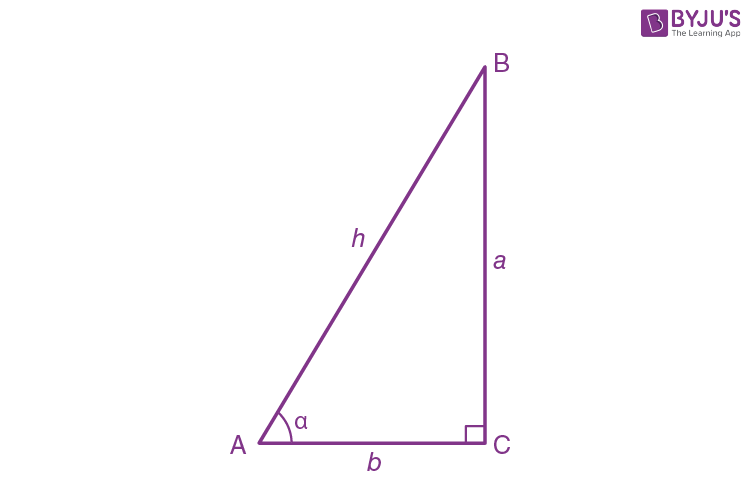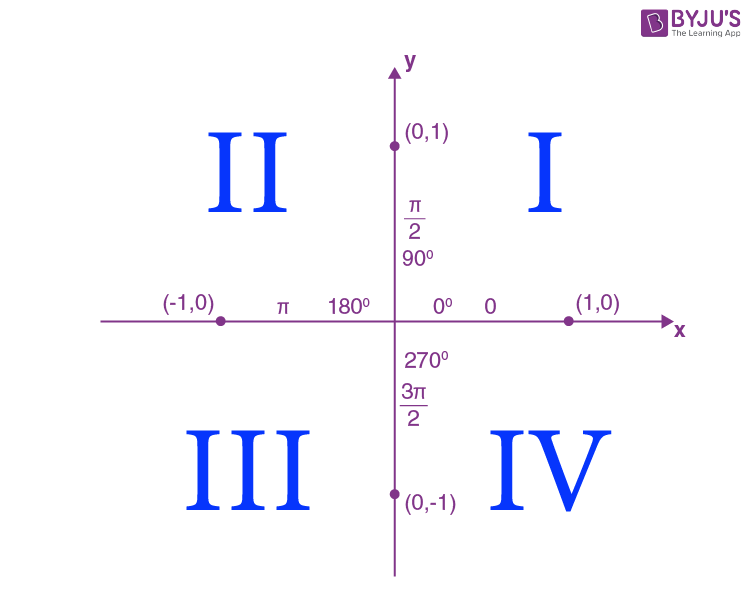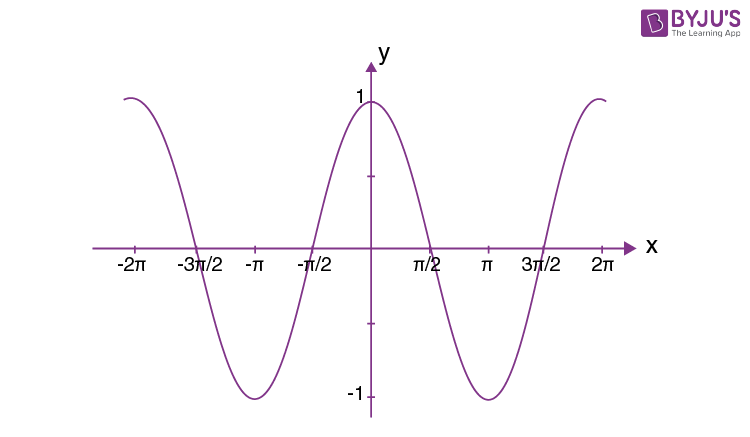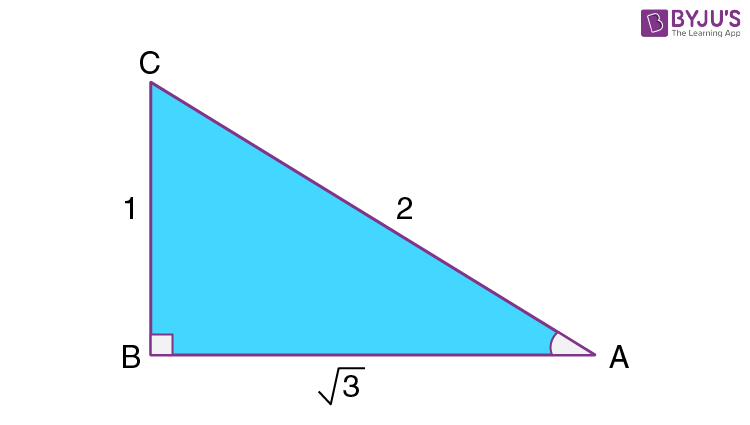# Cosine Function

The cosine function (or cos function) in a triangle is the ratio of the adjacent side to that of the hypotenuse. The cosine function is one of the three main primary trigonometric functions and it is itself the complement of sine(co+sine). There are various topics that are included in the entire cos concept. Here, the main topics that are focussed include:

• Cosine Definition
• Cosine Formula
• Cosine Table
• Cosine Properties With Respect to the Quadrants
• Cos Graph
• Inverse Cosine (arccos)
• Cosine Identities
• Cos Calculus
• Law of Cosines in Trigonometry
• Cosine Worksheet
• Trigonometry Related Articles for Class 10
• Trigonometry Related Articles for Class 11 and 12
• Other Trigonometry Related Topics

## Other Trigonometric Functions

 Sine Function Tan Function Cosec (Csc) Function Sec Function Cot Function

### Cosine Definition

In a right-triangle, cos is defined as the ratio of the length of the adjacent side to that of the longest side i.e. the hypotenuse. Suppose a triangle ABC is taken with AB as the hypotenuse and α as the angle between the hypotenuse and base.Now, for this triangle,

### Cosine Formula

From the definition of cos, it is now known that it is the adjacent side divided by the hypotenuse. Now, from the above diagram,

coα AC/AB

Or,

coα b/h

## Cosine Table

 Cosine Degrees Values cos 0° 1 cos 30° √3/2 cos 45° 1/√2 cos 60° 1/2 cos 90° 0 cos 120° -1/2 cos 150° -√3/2 cos 180° -1 cos 270° 0 cos 360° 1

### Cosine Properties With Respect to the Quadrants

It is interesting to note that the value of cos changes according to the quadrants. In the above table, it can be seen that cos 120°, 150° and 180° have negative values while cos 0°, 30°, etc. have positive values. For cos, the value will be positive in the first and the fourth quadrant.Degree Range Quadrant Cos Function Sign Cos Value Range 0°to 90° 1st Quadrant + (Positive) 0 < cos(x) < 1 90° to 180° 2nd Quadrant – (Negative) -1 < cos(x) < 0 180° to 270° 3rd Quadrant – (Negative) -1 < cos(x) < 0 270° to 360° 4th Quadrant + (Positive) 0 < cos(x) <1

### Cos Graph

The cosine graph or the cos graph is an up-down graph just like the sine graph. The only difference between the sine graph and the cos graph is that the sine graph starts from 0 while the cos graph starts from 90 (or π/2). The cos graph given below starts from 1 and falls till -1 and then starts rising again.## Arccos (Inverse Cosine)

The cos inverse function can be used to measure the angle of any right-angled triangle if the ratio of the adjacent side and hypotenuse is given. The inverse of sine is denoted as arccos or cos-1 x.

For a right triangle with sides 1, 2, and √3, the cos function can be used to measure the angle.In this, the cos of angle A will be, cos(a)= adjacent/hypotenuse.

So, cos(a) = √3/2

Now, the angle “a” will be cos−1(√3/2)

Or, a = π/6 = 30°

### Important Cos Identities

• cos(x) + sin(x) = 1
• cos θ = 1/sec θ
• cos (−θ) = cos (θ)
• arccos (cos (x)) = x + 2kπ  [where k=integer]
• Cos (2x) = cos(x) − sin(x)
• cos (θ) = sin (π/2 − θ)

Below, all the other trigonometric functions in terms of cos function are also given.

### Other Trigonometric Functions in Terms of Sine

 Trigonometric Functions Represented as Sine sin θ ±√(1-cos2θ) tan θ ±√(1-cos2θ)/cos θ cot θ ±cos θ/√(1-cos2θ) sec θ ±1/cos θ cosec θ ±1/√(1-cos2θ)

### Cos Calculus

For cosine function f(xcos(x), the derivative and the integral will be given as:

• Derivative of cos(x), f′ (x) = −sin (x)
• Integral of cos(x), ∫f (x) dx = sin(x) + C)      [where C is the constant of integration)

## Law of Cosines in Trigonometry

The law of cosine or cosine rule in trigonometry is a relation between the side and the angles of a triangle. Suppose a triangle with sides a, b, and c and with angles A, B, and C are taken, the cosine rule will be as follows.

According to cos law, the side “c” will be:

c= a+ b− 2ab cos (C)

It is important to be thorough with the law of cosines as questions related to it are common in the examinations.

### Also Check:

 Cos 1 Degree is 0.99 Cos 2 Degree is 0.99 Cos 5 Degree is 0.996 Cos 8 Degree is 0.990 Cos 10 Degree is 0.984 Cos 15 Degree is 0.965 Cos 20 Degree is 0.939 Cos 30 Degree is 0.866 Cos 40 Degree is 0.766 Cos 50 Degree is 0.642 Cos 70 Degree is 0.342 Cos 80 Degree is 0.173 Cos 100 Degree is -0.173 Cos 105 Degree is -0.258 Cos 210 Degree is -0.866 Cos 240 Degree is -0.5 Cos 270 Degree is 0 Cos 330 Degree is 0.866

### Cos Questions (Worksheets)

1. sin (cos-13/5)
2. In a triangle PQR, PR is 14 cm, QR is 10 cm, and angle RQP is 63 degrees. Calculate angle RPQ and the length of PQ.
3. In triangle ABC, AB is 6 cm, AC is 13 cm, and the angle CAB is 91 degrees. Calculate the length of BC.
4. Derive the value of cos 60 geometrically.
5. A ramp is pulled out of the back of a truck. There is a 38 degrees angle between the ramp and the pavement. The distance from the end of the ramp to the back of the truck is 10 feet. Calculate the length of the ramp?

### Trigonometry Related Articles for Class 11 and 12

Stay tuned with BYJU’S and get more trigonometry and Maths-related articles in a simple and detailed way. Also, register at BYJU’S to get access to 1000+ hours of engaging video lessons for different subjects and classes.

Quiz on Cosine Function